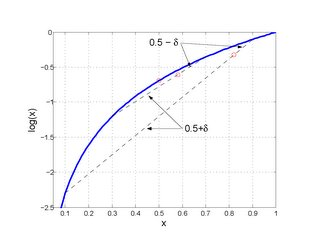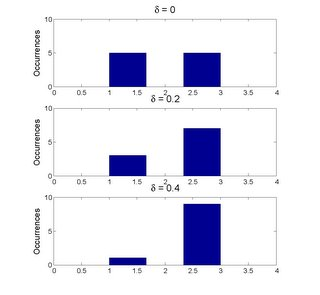## Friday, January 21, 2005

### Understanding Mutual Information

The mutual information between random variables, I(X,Y) is a measure of information overlap, i.e. I(X,Y) = H(X) - H(X|Y) = H(Y) - H(Y|X) where H() is a measure of entropy/information. Information is a nebulous concept so we define information here to be equivalent to entropy -- a functional of the probability density function of the random variable. Mutual information between random variables is then a probabilistic measure. It is a measure of the probability of relating the two variables regardless of the form of the relationship that might be applied. If the pdf of a random variable is not known, then the entropy must be estimated from an estimate of the pdf. A consistent and straightforward method of estimating the entropy functional is by substitution of the pdf estimate for the pdf in the entropy function. In this case, the estimation of the probability of relating variables is dependent directly on the statistical properties of the joint pdf estimate of the r.v.s.

If we are without any knowledge of the random variables that might be used to form the estimate of their pdf, the frequentist method of estimation is all that is available. The relative number of occurrences of an observation of the variables is the estimate of the probability of that event over those measurement values. The grouping of occurrences can be done several ways typically either by kernels or by histograms. Again, without a priori knowledge of the r.v.s, we favor uniform histograms for their computationally efficiency. The drawback of histograms is that they enforce a discrete partition on the observations regardless of whether the underlying process that generated the r.v.s is discrete or not. However, when the dimensionality of the data is even moderately great and the number of observations is fixed, any benefit in the use of smooth kernels in approximating the pdf of a continuous process is negligible. Since we envision a locally smooth method of reconstruction of the process relationship based on the local estimates of MI, the discreteness of histograms is not a primary concern.

The partitioning of the variable space by bins gives us a basis for making local relations between the variables (assuming a causal relation we will label these as 'input' and 'output'). Ideally, a local bijection exists between each input and output bin. A necessary and sufficient condition for this existence is that the joint probability of the input/output bin in the input/output space is equal to its marginal probability in both the input and output spaces. Of course, a bijection may not exist between the events that fall into the bin, only the bins themselves.

Entropy is the (negative of the) expectation of the logarithm of the pdf of a r.v. Unlike linear measures of concentration, the logarithm used in the entropy functional has the effect of disproportionately weighting the differences in a distribution between bins so that the closer to equivalency the distribution of probability mass between bins, the lesser the change in entropy. Conversely, small deviations from concentrations of probability mass lead to disproportionately greater differences in entropy.Note that the linear distributive probability relation demonstrated here is unique to the logarithm among other functions with the property previously mentioned.For continuous processes, the relation between joint and marginal partitions will tend to be symmetric in the joint space. In general, the mutual information between two random variables can be visualized as the degree to which the probability masses of the partitions in of one marginal space can be traced through the joint space to the marginal distribution of the other variable. The more certain this can be done, the stronger and more accurate will be the mapping between the divisions of the variables. This is then the basis for a global mapping between the variables that is statistically robust and measurably accurate.